Constructing angles - 60, 90, 45, 30 and others

Chapter 11 Class 9 Constructions
Concept wise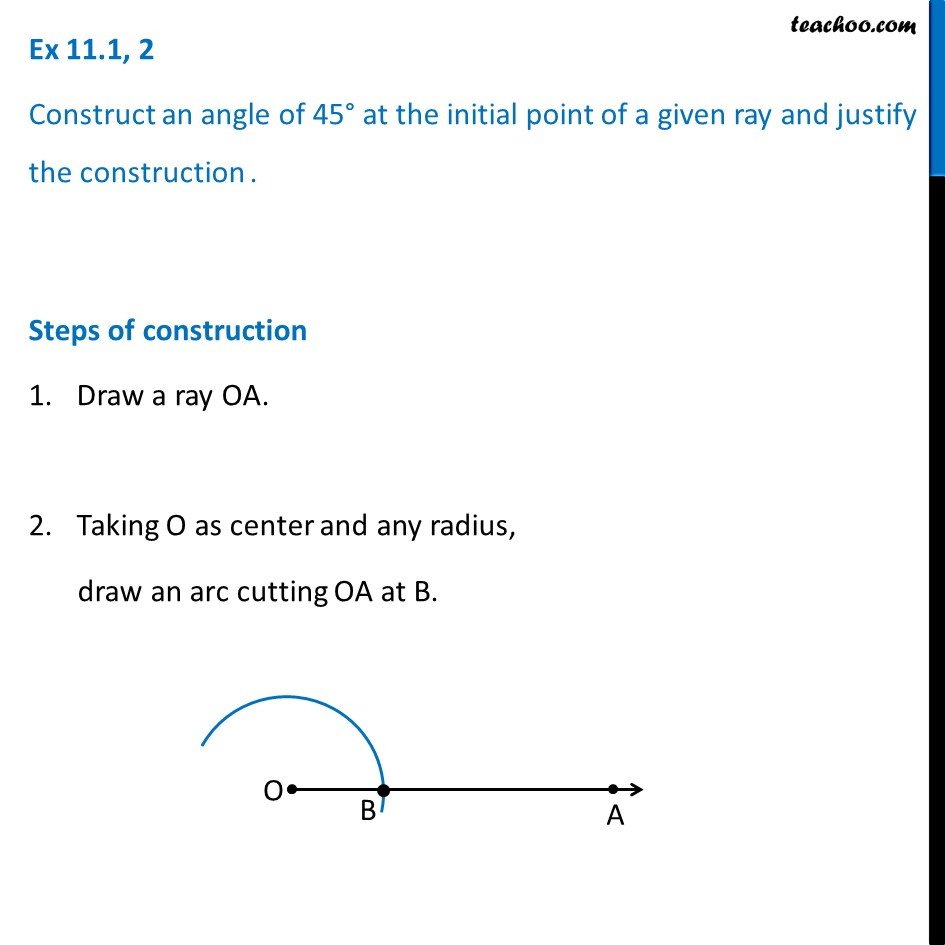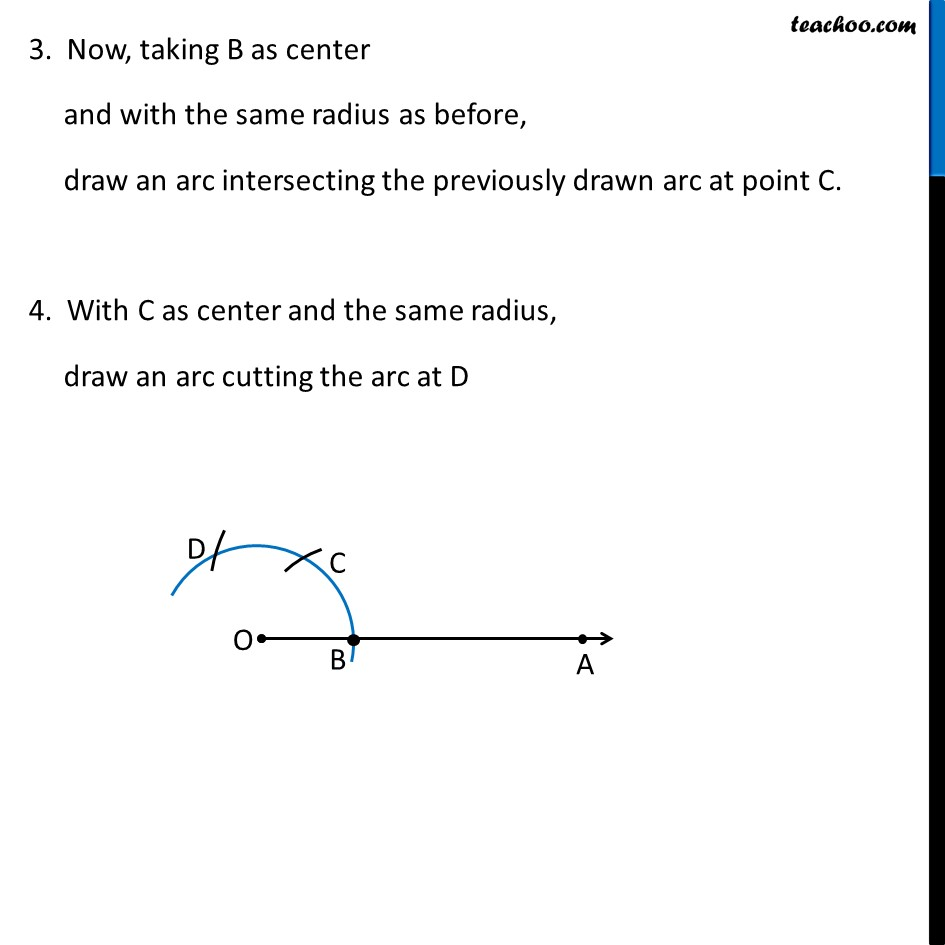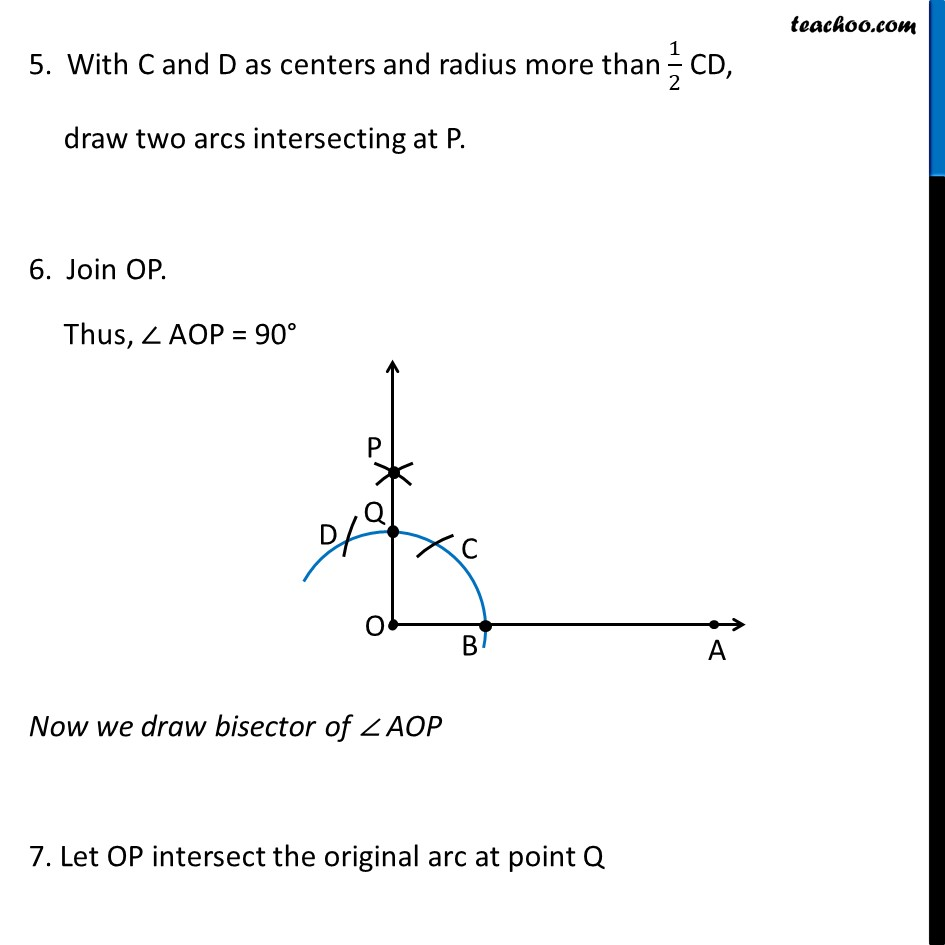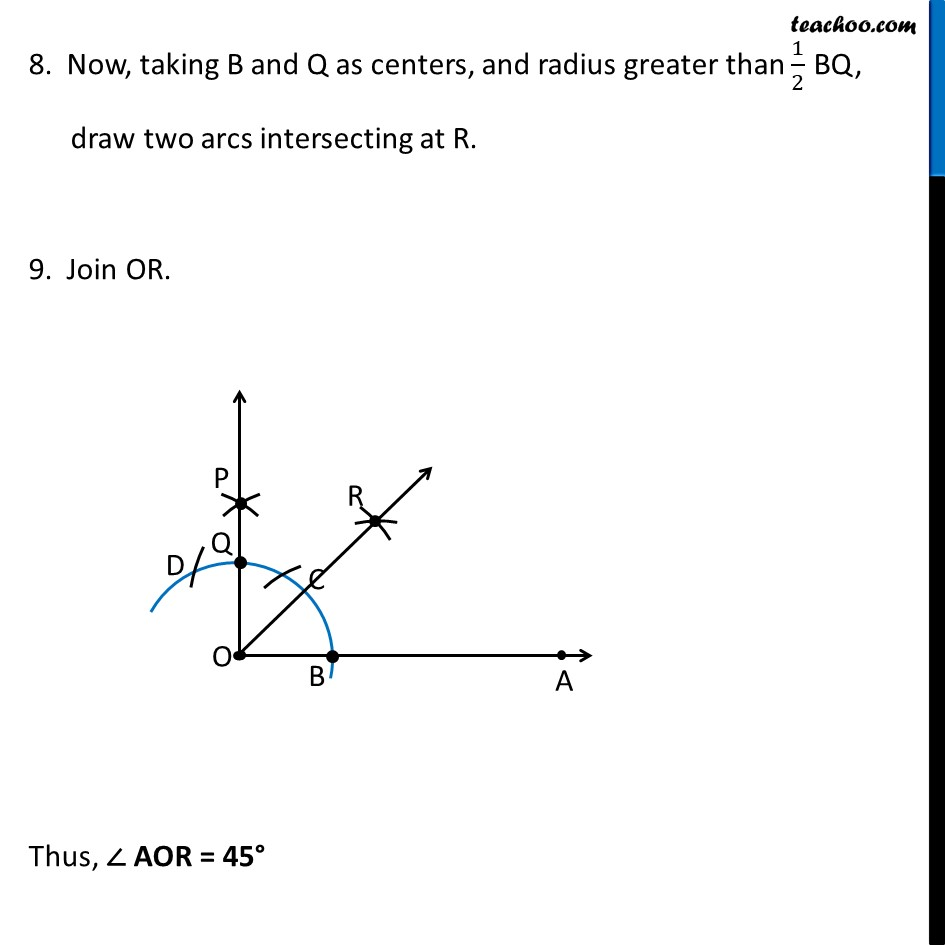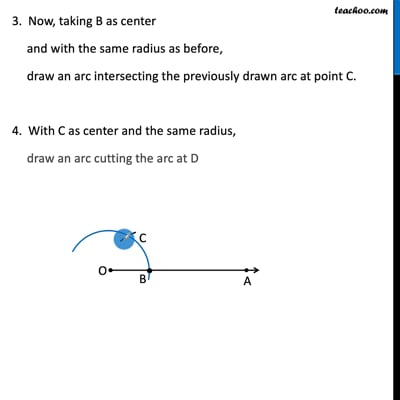This video is only available for Teachoo black users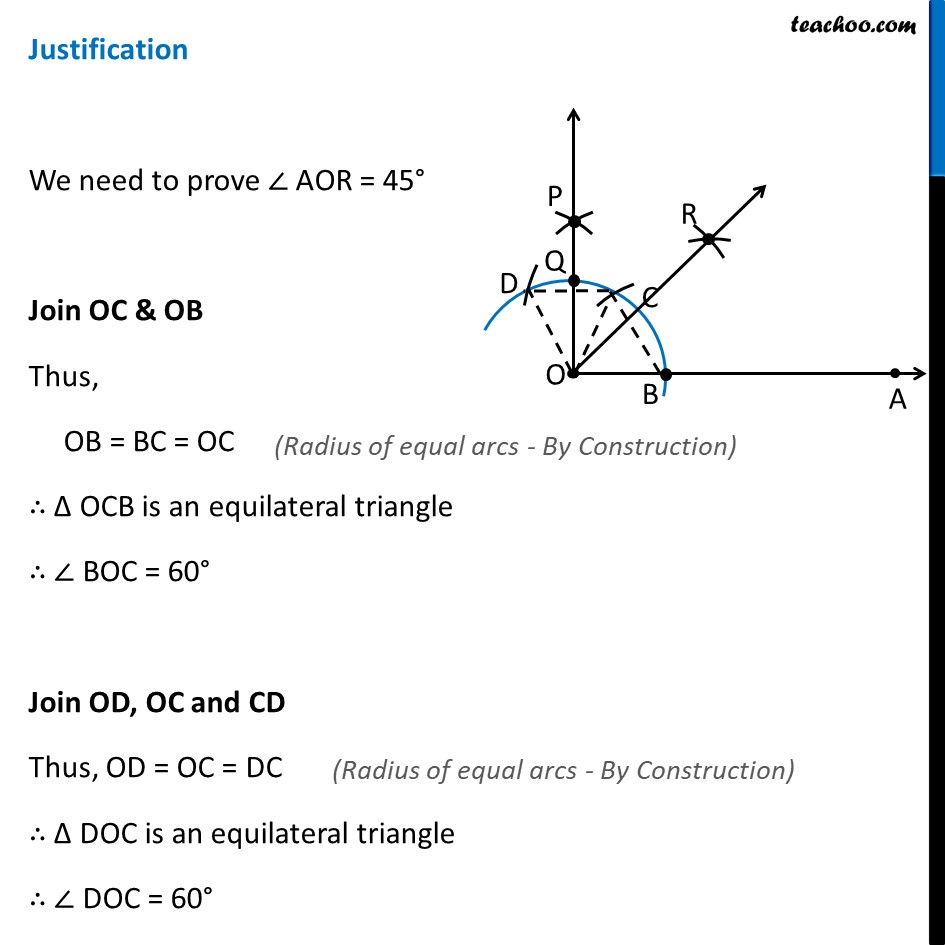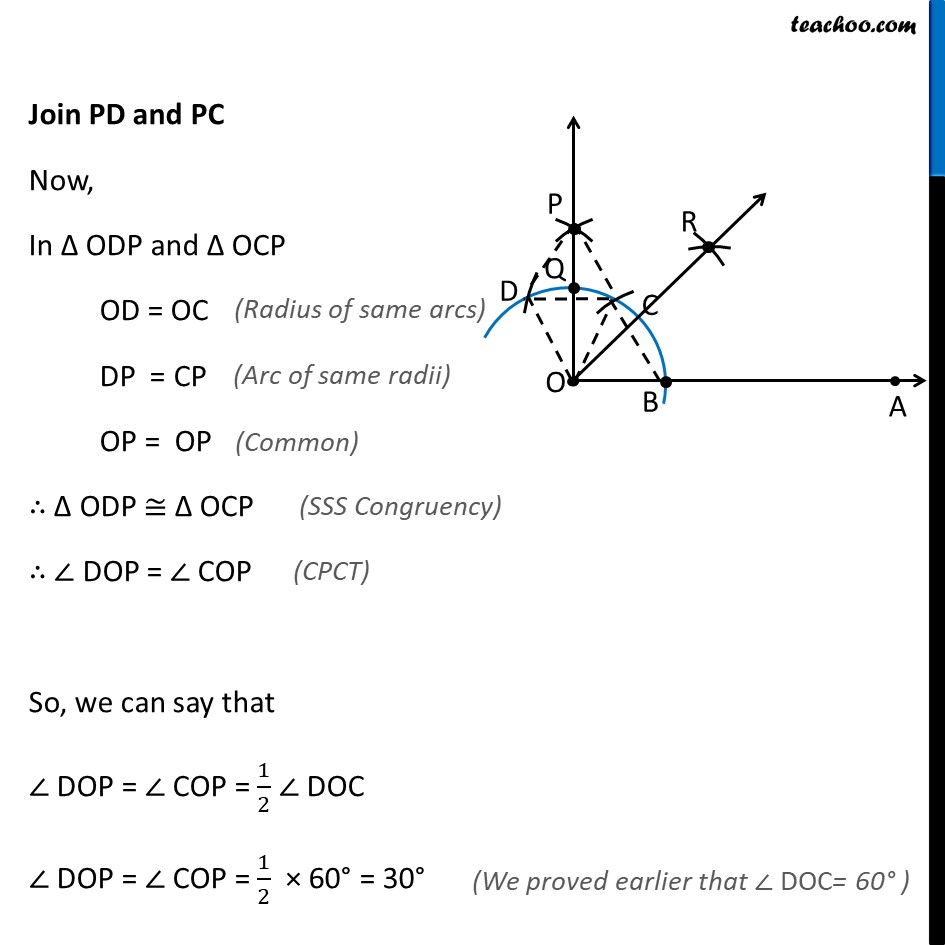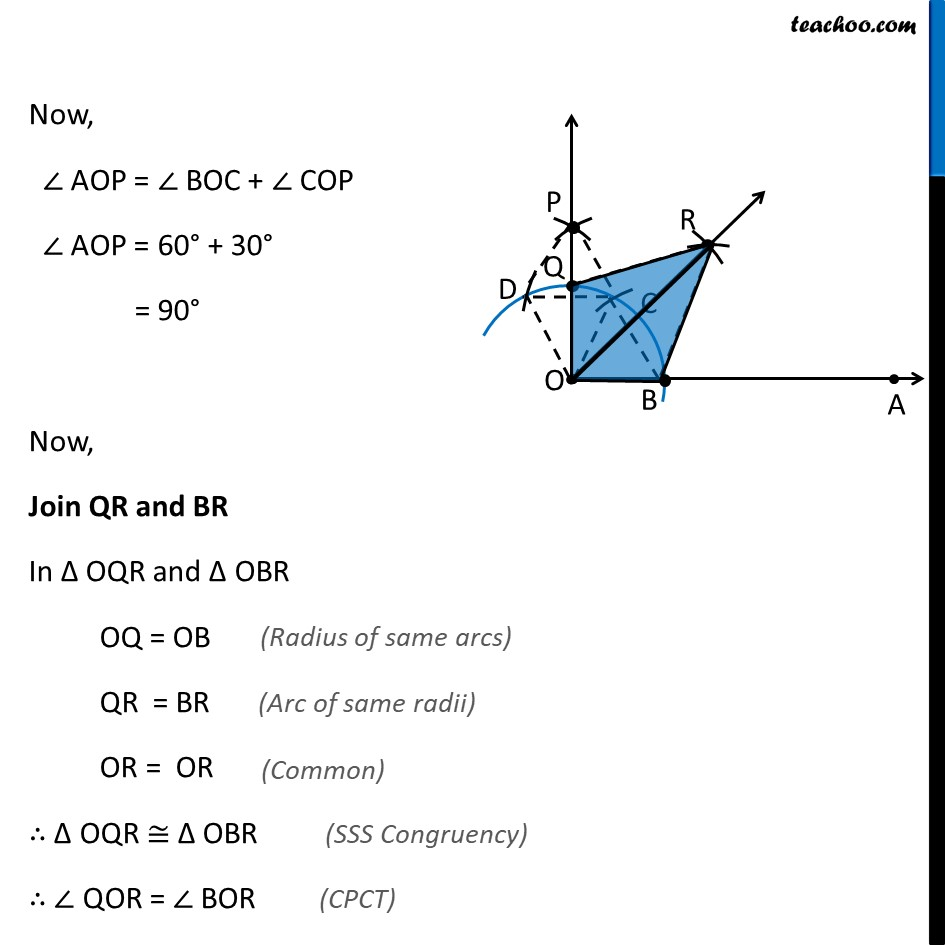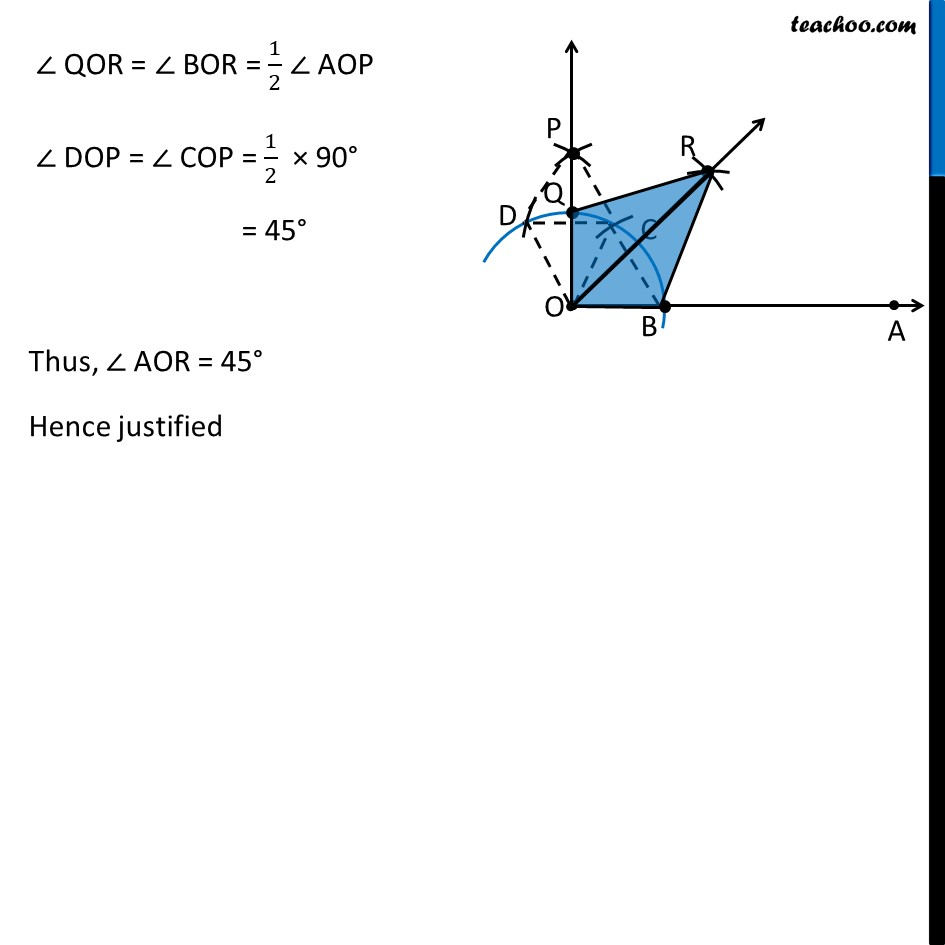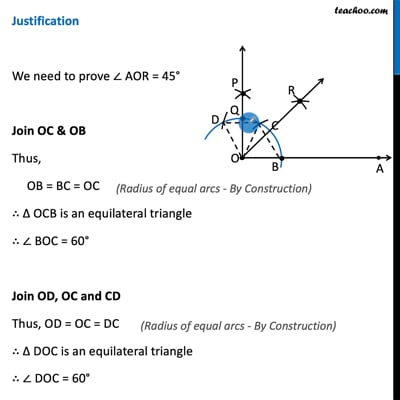This video is only available for Teachoo black users

Introducing your new favourite teacher - Teachoo Black, at only ₹83 per month

### Transcript

Ex 11.1, 2 Construct an angle of 45° at the initial point of a given ray and justify the construction . Steps of construction Draw a ray OA. Taking O as center and any radius, draw an arc cutting OA at B. 3. Now, taking B as center and with the same radius as before, draw an arc intersecting the previously drawn arc at point C. 4. With C as center and the same radius, draw an arc cutting the arc at D 5. With C and D as centers and radius more than 1/2 CD, draw two arcs intersecting at P. 6. Join OP. Thus, ∠ AOP = 90° Now we draw bisector of ∠ AOP 7. Let OP intersect the original arc at point Q 8. Now, taking B and Q as centers, and radius greater than 1/2 BQ, draw two arcs intersecting at R. 9. Join OR. Thus, ∠ AOR = 45° Justification We need to prove ∠ AOR = 45° Join OC & OB Thus, OB = BC = OC ∴ Δ OCB is an equilateral triangle ∴ ∠ BOC = 60° Join OD, OC and CD Thus, OD = OC = DC ∴ Δ DOC is an equilateral triangle ∴ ∠ DOC = 60° Join PD and PC Now, In Δ ODP and Δ OCP OD = OC DP = CP OP = OP ∴ Δ ODP ≅ Δ OCP ∴ ∠ DOP = ∠ COP So, we can say that ∠ DOP = ∠ COP = 1/2 ∠ DOC ∠ DOP = ∠ COP = 1/2 × 60° = 30° (Radius of same arcs) (Arc of same radii) (Common) (SSS Congruency) (CPCT) (We proved earlier that ∠ DOC= 60° ) Now, ∠ AOP = ∠ BOC + ∠ COP ∠ AOP = 60° + 30° = 90° Now, Join QR and BR In Δ OQR and Δ OBR OQ = OB QR = BR OR = OR ∴ Δ OQR ≅ Δ OBR ∴ ∠ QOR = ∠ BOR (Radius of same arcs) (Arc of same radii) (Common) (SSS Congruency) ∠ QOR = ∠ BOR = 1/2 ∠ AOP ∠ DOP = ∠ COP = 1/2 × 90° = 45° Thus, ∠ AOR = 45° Hence justified